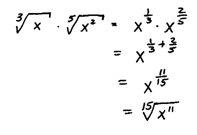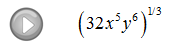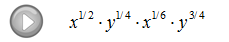## Algebra

Showing posts with label denominator. Show all posts
Showing posts with label denominator. Show all posts

### Rational Exponents

Rational (fractional) exponents, indicate that we have a radical expression.

They are intimidating at first but you will quickly get used to working with them. The denominator is the index and the numerator is the exponent.[ Video Instruction: Rational Exponents ]

Rewrite the radical expression using rational exponents.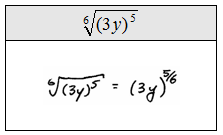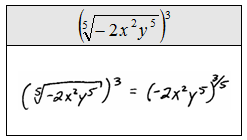Convert to a radical and then simplify.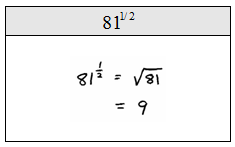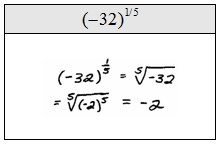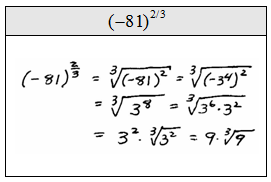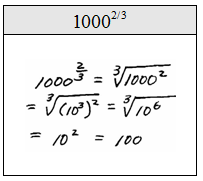When simplifying, you can use the rules of exponents to simplify or you can convert to a radical and then simplify. Both methods yield the same result.

Assume all variables are positive and use the rules of exponents to simplify.Simplify. (Assume all variables are positive.)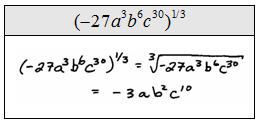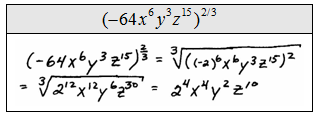Notice that all of the above problems worked out nicely because the exponents were multiples of the root.  This is not always the case as illustrated below.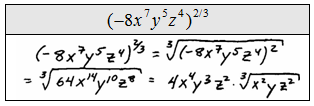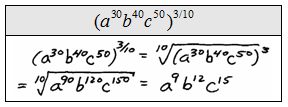A quick way to simplify radicals is to divide the index into the exponents. This will tell us what the exponent of the base should be outside the radical and the remainder will be the exponent of the base inside the radical. All the rules of exponents hold true when working with rational exponents.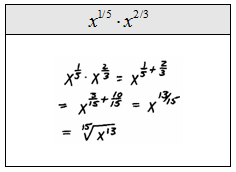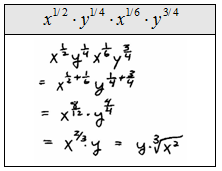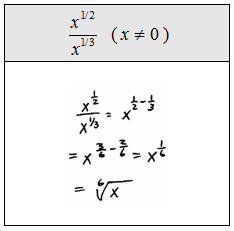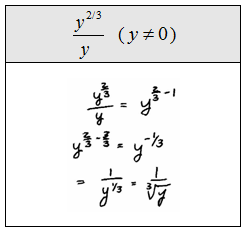Sometimes we will be asked to multiply radicals with different indices.  This may seem impossible at first, but it can be done using the rules of exponents as follows.